# Machine Learning For Stock Price Prediction Using Regression

The other day I was reading an article on how AI and machine learning have progressed so far and where they are going. I was awestruck and had a hard time digesting the picture the author drew on possibilities in the future.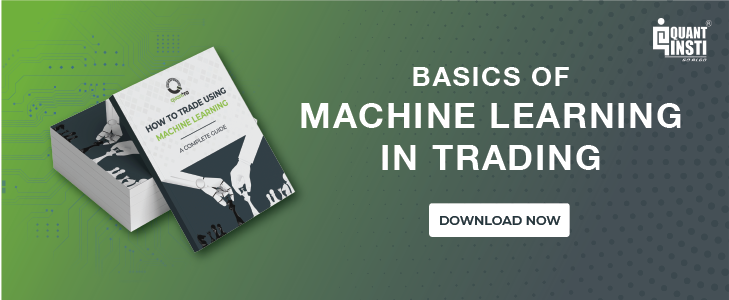Here is how I reacted. (No, I am not as good looking as Joey but you get the idea)And here is one of the possibilities where AI could be applied in medical field, para from the article,

A surgeon could control a machine scalpel with her motor cortex instead of holding one in her hand, and she could receive sensory input from that scalpel so that it would feel like an 11th finger to her. So it would be as if one of her fingers was a scalpel and she could do the surgery without holding any tools, giving her much finer control over her incisions. An inexperienced surgeon performing a tough operation could bring a couple of her mentors into the scene as she operates to watch her work through her eyes and think instructions or advice to her. And if something goes really wrong, one of them could “take the wheel” and connect their motor cortex to her outputs to take control of her hands.

You can read the article here.

At this moment, AI and Machine Learning have already progressed enough so can we now apply these machine learning techniques in trading and achieve a great level of accuracy

### What is Machine Learning?

The definition is this, “Machine Learning is where computer algorithms are used to autonomously learn from data and information and improve the existing algorithms”

But in simple terms, Machine learning is like this, take this kid for example - consider that he is an intelligent machine, now,

1. Give him a chess board
2. Explain the basic rules of the game
3. Give records of say 100 good games
4. Lock the kid in a room (throw in some food and water as well)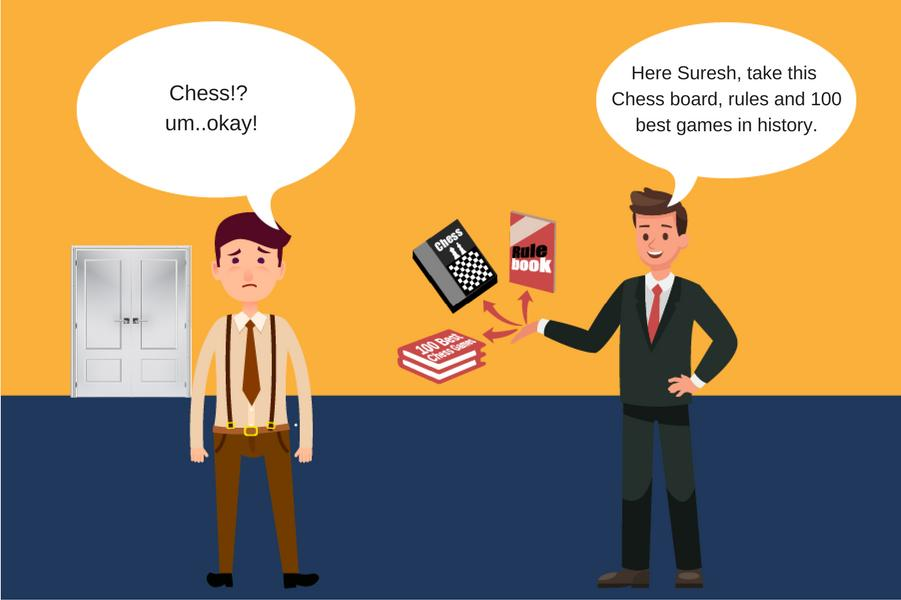10 days later,When the kid walks out of that room, you will be looking at a pretty good chess player. In this case - the kid is the machine, past game records are the data and chess rule book is the algorithm. We only fed a basic algorithm to the machine and some data to learn from. The machine sipped through the data, understood which moves improved the chances of winning the game and added those moves to the algorithm. That is the whole concept of Machine Learning. The advantage in case of computers compared to humans is that computers can do this quickly, for bigger data sets and for a continuous period of time.

However, that’s just one example, there are different aspects of Machine Learning and they’re darn interesting. But we’ll stick to the basics in this post.

Also, people often get confused between Artificial Intelligence, Machine Learning, and Deep Learning. AI is a much larger space covering a lot of things, whereas machine learning is a part of AI and further Deep Learning is a subset of Machine learning. Here, I have hand drawn this diagram for you.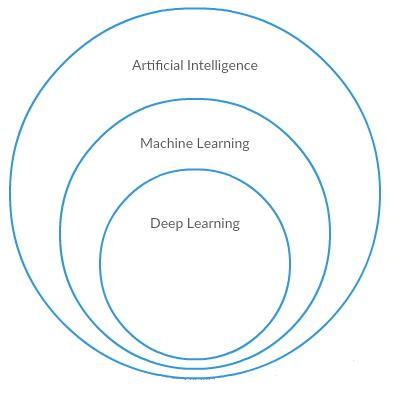If you want to dive deeper into specifics of these topics you can check out this.

### Why has Machine Learning become such a buzz word lately?

If you dig deeper, you’d find that Machine Learning has been around since long. For example, in 1763, Thomas Bayes published a work ‘An Essay towards solving a Problem in the Doctrine of Chances’ which lead to ‘Bayes Rule’, one of the important algorithms used in Machine Learning

But today, Machine Learning is advancing at an unprecedented speed. We might not realize it but applications of Machine Learning are everywhere, for example,

• Recommendation systems (facebook news feed, amazon product recommendation)
• Natural language processing (Siri, google voice)
• Medical diagnosis (spotting patterns in images)
• Object recognition and tracking (facial recognition, license plate reading, and tracking)
• Mining 'Big Data' - Analytics (stock with this pattern tend to go up)
• Classification and Clustering of data (fraud detection, sequence mining etc.)

All of these things are based on the concept of learning from the past data and predicting the outcome for an unseen/new situation, the same way humans learn. But the advantage for computers is that they can process data at a much larger scale and with much larger complexity, something that is simply incomprehensible to humans.

Given today’s environment where you have trillions of gigabytes of data being generated every day, it just becomes impossible for humans to process and make useful inferences out of it. Sure, smart people might be able to make better predictions and inferences but Machine learning algorithms beat us at the scale and complexity level. And over time the predictions made by these computers will surpass the human level.

So when every industry has started implementing Machine Learning in some form or the other, why shouldn’t you as a trader use this to your advantage to upgrade your trading skills. Guess what? Machine Learning and trading goes hand-in-hand like cheese and wine. Some of the top traders and hedge fund managers have used machine learning algorithms to make better predictions and as a result money!

In this post, I will teach you how to use machine learning for stock price prediction using regression.

### What is Linear Regression?

Here is the formal definition, “Linear Regression is an approach for modeling the relationship between a scalar dependent variable y and one or more explanatory variables (or independent variables) denoted X” 

Let me explain the concept of regression in a very basic manner, so imagine that you run a company that builds cars and you want to understand how the change in prices of raw materials (let’s say Steel) will affect the sales of the car. The general understanding is this, the rise in the price of steel will lead to a rise in the price of the car resulting in lesser demand and in turn lesser sales. But how do we quantify this? And how do we predict how much change in sales will happen based on the degree of change in steel price. That’s when the regression comes into the picture.

Let’s consider the below-mentioned sample data for understanding,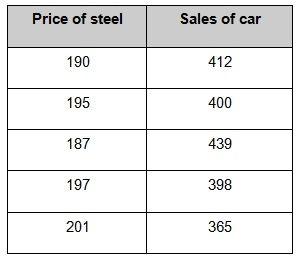Let’s put this into a graph, this graph is called as scatterplot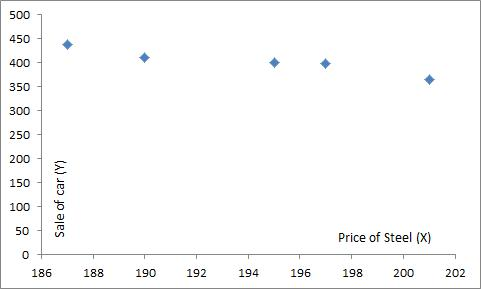Y axis is the sales of a car (this is our dependent variable) and X axis is the price of steel (independent variable). By general observation, you can tell that whenever there is a drop in steel prices the sales of the car improves.

The sample data is the training material for the regression algorithm. And now it will help us in predicting, what kind of sales we might achieve if the steel price drops to say 168 (considerable drop), which is a new information for the algorithm.

We will take Excel’s help in crunching the numbers,

So when you put the sample data in an excel spreadsheet and perform regression (you can see this video to learn how to perform regression in excel), you will get the below shown regression line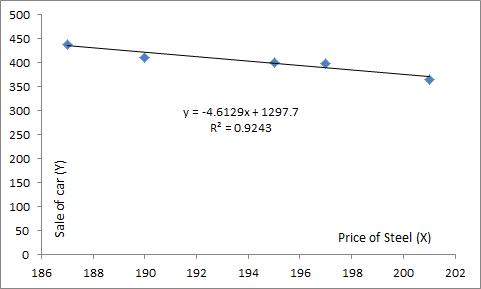And some weird looking numbers like these, but for basic understanding, I will only focus on a few metrics in this. The purpose of the linear regression function is to find a line that is closest from all data points so that whenever we want to calculate the prediction for a new dependent variable we can pick the subsequent point on the line corresponding to the independent variable on X axis.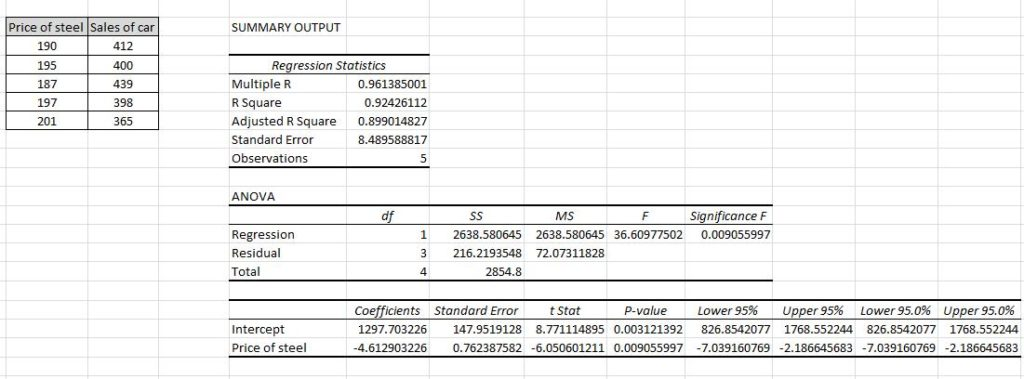So the above calculations were done based on the equations below, also called as regression expression.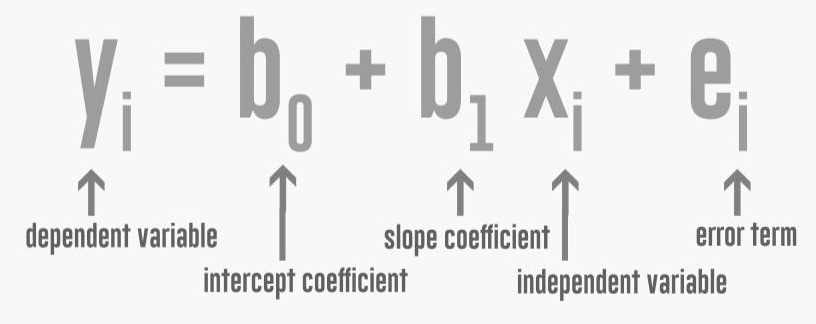‘Y’ - Sales of the car or dependent variable, this is what we are trying to predict

‘X’ - Price of steel or independent variable, this will be used to predict ‘Y’

‘b0’-  Intercept is the value at which our regression line crosses the ‘y’ axis

‘b1’ - Slope coefficient, this tell us the amount of change in y that can be expected to result from a unit increase in x

‘ei’ -  Error term, when the relationship we express using this equation for any variable does not fully represent the actual relationship between the independent variable and the dependent variable, the variable representing this difference is known as error term or the residual, disturbance or remainder term

R2 - R squared or coefficient of correlation, this shows how close the data is to the fitted regression line

If you look at the regression graph above, you will see a regression equation, which is

y = -4.6129x + 1297.7

So in this equation,

b1 = -4.6129

b0 + ei = 1297.7

Do notice that slope coefficient or b1 is negative, this means that the two variables (steel price and sale of car) are negatively correlated, meaning when the price of steel rises the sale of car drops.

R2 of the equation is 0.92 which is good, we want this value to be as close to 1 as possible for better predictions.

So now coming to the awesome part, take any change in the price of Steel, for example price of steel is say 168 and we want to calculate the predicted rise in the sale of cars. Here’s how you do it,

(sales of car) = -4.6129 x (168) + 1297.7

Sale of car = 522.73 when steel price drops to 168

Isn’t that amazing? Guess what even if there were multiple variables that affected the sales of a car (as there are in the real world) we would be able to calculate a prediction. When there are more than one independent variables in regression it is called as Multiple Regression Model.

I hope you understood this part if you still have any queries you can ask them to our community here

### Regression and Stock Market

Now, let me show you a real life application of regression in the stock market. For example, we are holding Canara bank stock and want to see how changes in Bank Nifty’s (bank index) price affect Canara’s stock price. Our aim is to find a function that will help us predict prices of Canara bank based on the given price of the index.

We will take Bank Nifty & Canara’s close prices for last 2 months, we are taking adjusted close prices for data consistency. Please note, having accurate data is very important, as even one of the numbers in the data can cause the regression function to change significantly.

Out of this data we will treat first 40 days as training data and last 20 days as the test data, wherein we will check how close the predictions made by the regression algorithm are to the actual numbers.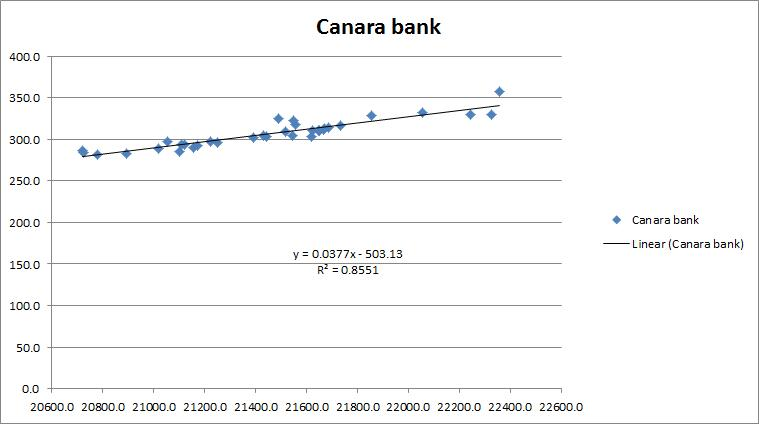The scatterplot shows the data. Using the same excel function we have drawn this regression line which has a coefficient of determination(R2) of 0.85. This means Canara Bank and Bank Nifty are 85% correlated.

Here is the regression expression,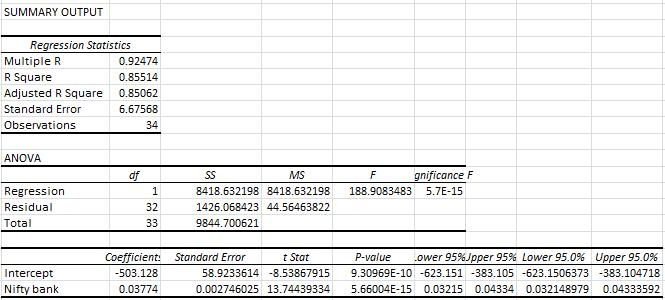Let’s look at the predictions made by the machine learning regression algorithm, the predictions are marked in blue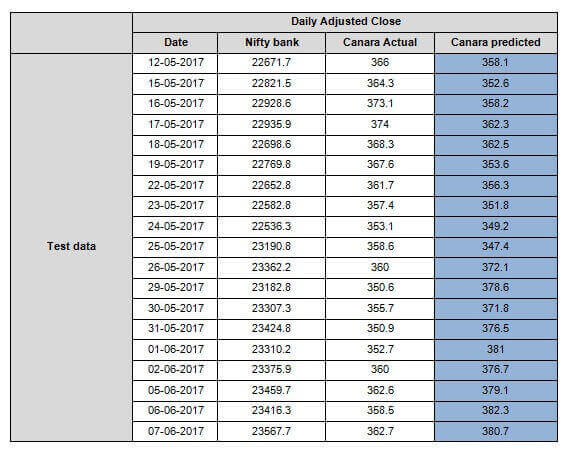Looking at the data, we can see the predictions are quite close (considering 85% coefficient), maybe not tradable but this gives us a direction. You can and should further improve this method by adding more than one independent variables. Doing so will help reduce the residual or error and help to get you closer to the actual price.

I have only taken 2 months data, you can take years of data for more accurate results. More the training data better the outcome. As you go on adding new market data to this you will see the function will keep improving itself by recalculating coefficient and intercept values.

### Next steps

Sign up for our latest course on ‘Neural Networks in Trading‘ on Quantra. This course is authored by Dr. Ernest P. Chan and covers core concepts such as back and forward propagation to using LSTM models in Keras, everything is covered in a simplified manner with additional reading material provided for advanced learners. You can also leverage from hands-on coding experience and downloadable strategies to continue learning post course completion.

Disclaimer: All data and information provided in this article are for informational purposes only. QuantInsti® makes no representations as to accuracy, completeness, currentness, suitability, or validity of any information in this article and will not be liable for any errors, omissions, or delays in this information or any losses, injuries, or damages arising from its display or use. All information is provided on an as-is basis.